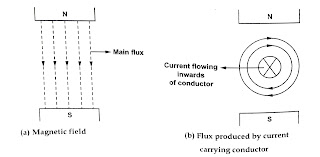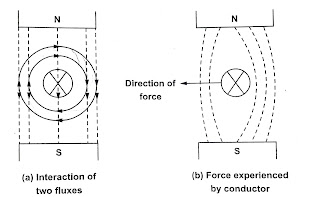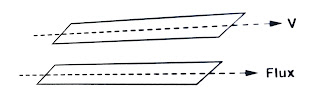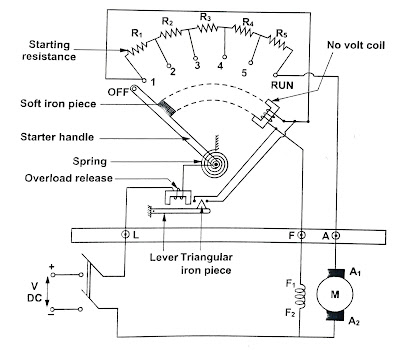#### INTRODUCTION

DC Motor is a device which converts an electrical energy into mechanical venergy. The input electrical energy is supplied by a DC supply. The construction of a DC machine is same whether it is a motor or a generator.

#### PRINCIPLE OF OPERATION OF A DC MOTOR

“When a current carrying conductor is placed in a magnetic field it experiences a mechanical force”. In a practical DC motor the required magnetic field is produced by field winding while armature conductor play the role of current carrying conductor and these armature conductors which are placed in the slots on the periphery experience a twisting or tuning force on the armature which is called torque. The torque is the product of force and the radius at which the force acts. In other words the twisting or turning force about an axis is called torque. Due to this torque in armature the motor starts rotating. Let us consider a single conductor placed in a magnetic fieldWhen a DC supply is given to the field pole a magnetic field is produced which is the main flux. Now the conductor considered is also excited by a separate DC supply which carries current in particular direction. So any conductor carrying current produces its own flux around it and the direction can be determined by right hand thumb rule.Now we have two fluxes present the main flux and flux produced by current carrying conductor. we can see that in one half of the conductor the direction of flux is same so gathering of flux occur. On the other half of the conductor the two fluxes opposes each other so weakening of flux occurs. Thus there exists a mechanical force on the conductor which acts from high flux density area to low flux density area. The magnitude of the force experienced by the conductor in a motor is given by

F = BlIN

B – Flux density

l – Length of conductor

I -Magnitude of current passing through conductor The direction of rotation of the motor can be determined by Fleming’s left hand rule.

See More:

See More:

See More: Four and Two Point Starter